Fixed Parameter Complexity Algorithms and Networks Fixed parameter

• Slides: 84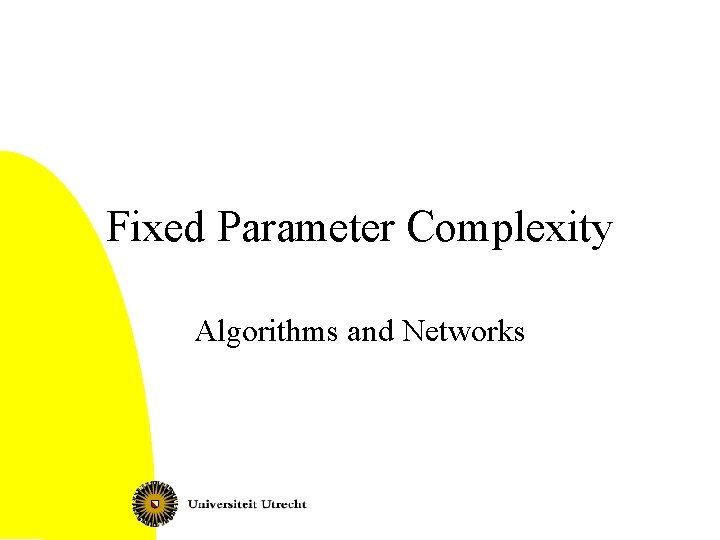Fixed Parameter Complexity Algorithms and Networks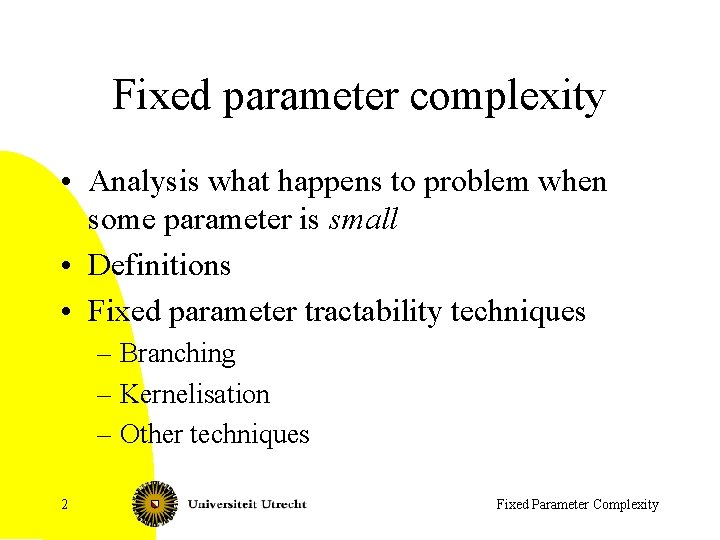Fixed parameter complexity • Analysis what happens to problem when some parameter is small • Definitions • Fixed parameter tractability techniques – Branching – Kernelisation – Other techniques 2 Fixed Parameter ComplexityMotivation • In many applications, some number can be assumed to be small – Time of algorithm can be exponential in this small number, but should be polynomial in usual size of problem 3 Fixed Parameter Complexity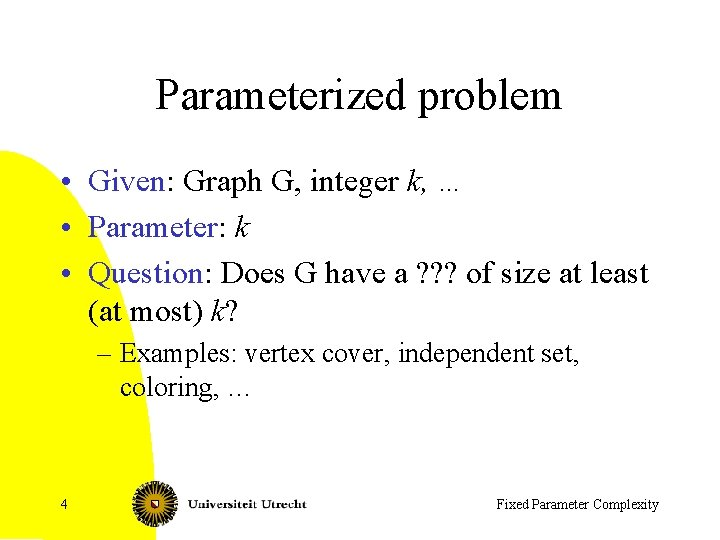Parameterized problem • Given: Graph G, integer k, … • Parameter: k • Question: Does G have a ? ? ? of size at least (at most) k? – Examples: vertex cover, independent set, coloring, … 4 Fixed Parameter ComplexityExamples of parameterized problems (1) Graph Coloring Given: Graph G, integer k Parameter: k Question: Is there a vertex coloring of G with k colors? (I. e. , c: V ® {1, 2, …, k} with for all {v, w}Î E: c(v) ¹ c(w)? ) • NP-complete, even when k=3. 5 Fixed Parameter Complexity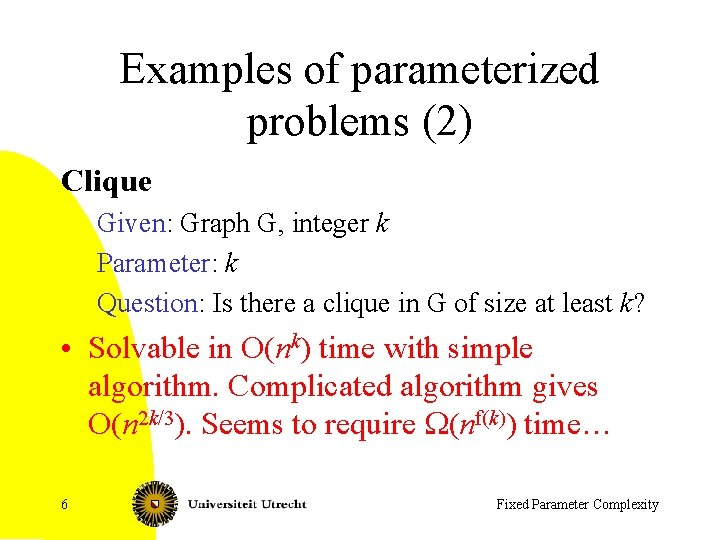Examples of parameterized problems (2) Clique Given: Graph G, integer k Parameter: k Question: Is there a clique in G of size at least k? • Solvable in O(nk) time with simple algorithm. Complicated algorithm gives O(n 2 k/3). Seems to require W(nf(k)) time… 6 Fixed Parameter ComplexityExamples of parameterized problems (3) Vertex cover Given: Graph G, integer k Parameter: k Question: Is there a vertex cover of G of size at most k? • Solvable in O(2 k (n+m)) time 7 Fixed Parameter Complexity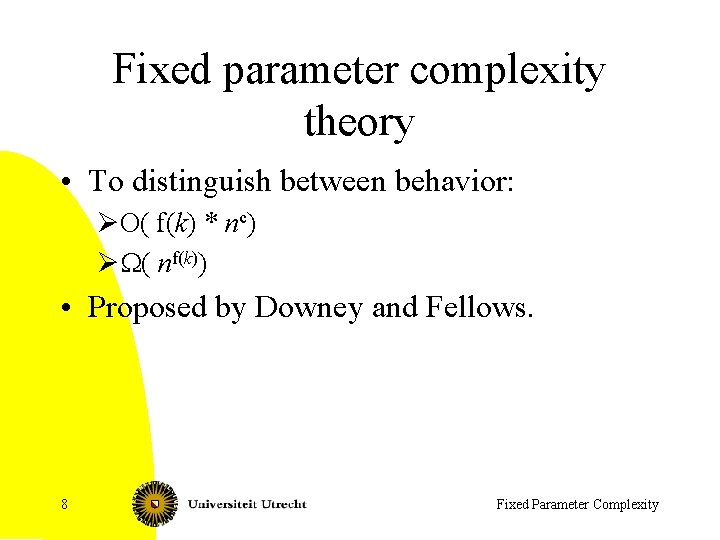Fixed parameter complexity theory • To distinguish between behavior: ØO( f(k) * nc) ØW( nf(k)) • Proposed by Downey and Fellows. 8 Fixed Parameter ComplexityParameterized problems • Instances of the form (x, k) – I. e. , we have a second parameter • Decision problem (subset of {0, 1}* x N ) 9 Fixed Parameter Complexity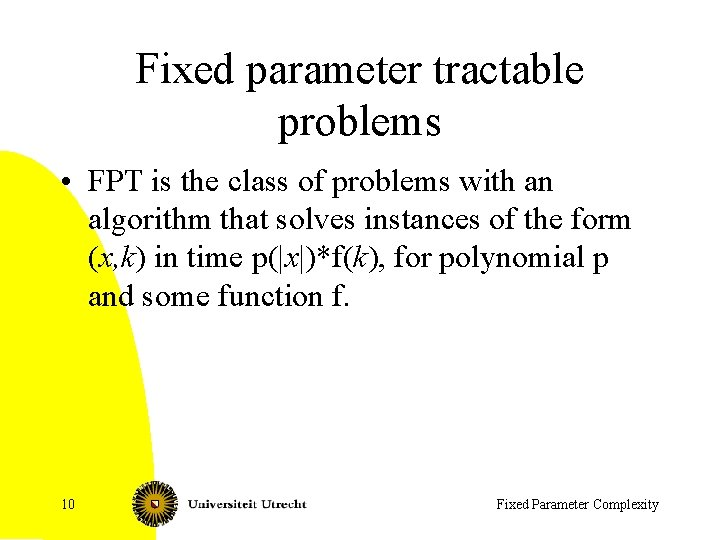Fixed parameter tractable problems • FPT is the class of problems with an algorithm that solves instances of the form (x, k) in time p(|x|)*f(k), for polynomial p and some function f. 10 Fixed Parameter Complexity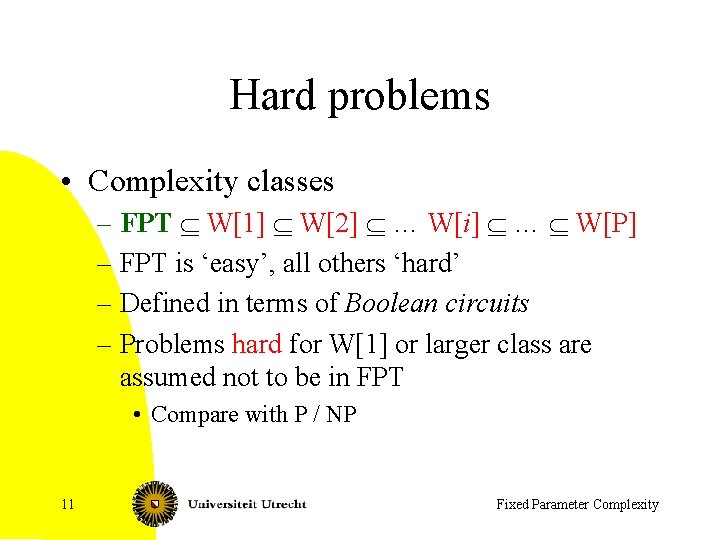Hard problems • Complexity classes – FPT Í W Í W Í … W[i] Í … Í W[P] – FPT is ‘easy’, all others ‘hard’ – Defined in terms of Boolean circuits – Problems hard for W or larger class are assumed not to be in FPT • Compare with P / NP 11 Fixed Parameter Complexity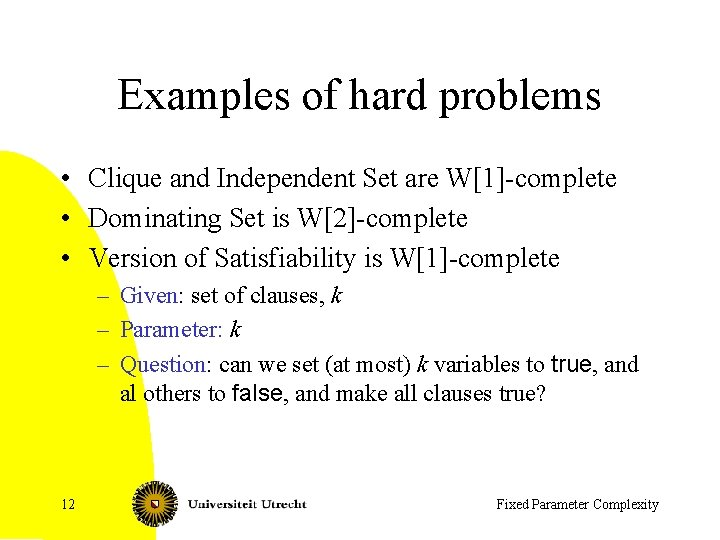Examples of hard problems • Clique and Independent Set are W-complete • Dominating Set is W-complete • Version of Satisfiability is W-complete – Given: set of clauses, k – Parameter: k – Question: can we set (at most) k variables to true, and al others to false, and make all clauses true? 12 Fixed Parameter Complexity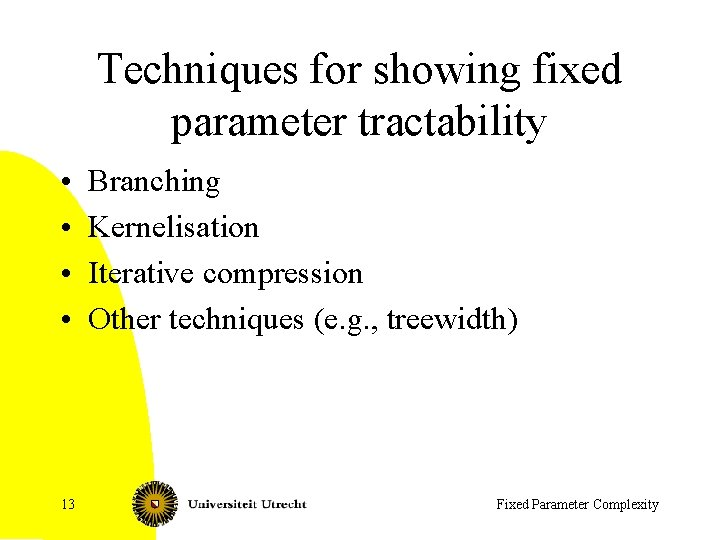Techniques for showing fixed parameter tractability • • 13 Branching Kernelisation Iterative compression Other techniques (e. g. , treewidth) Fixed Parameter ComplexityA branching algorithm for vertex cover • Idea: – Simple base cases – Branch on an edge: one of the endpoints belongs to the vertex cover • Input: graph G and integer k 14 Fixed Parameter Complexity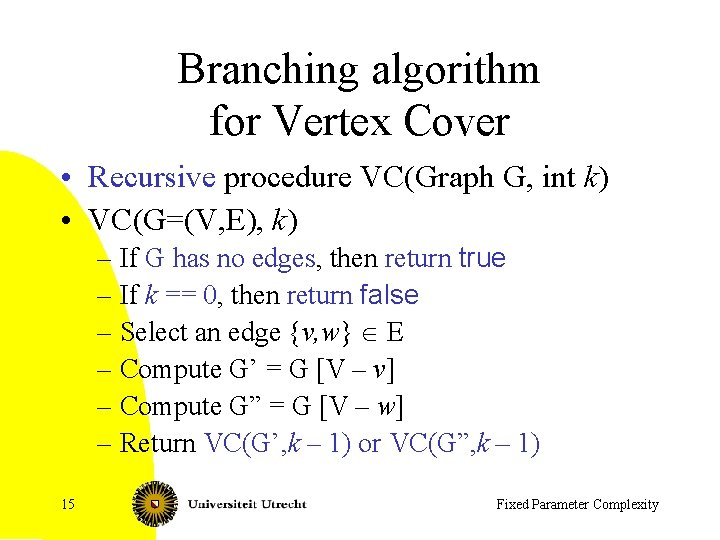Branching algorithm for Vertex Cover • Recursive procedure VC(Graph G, int k) • VC(G=(V, E), k) – If G has no edges, then return true – If k == 0, then return false – Select an edge {v, w} Î E – Compute G’ = G [V – v] – Compute G” = G [V – w] – Return VC(G’, k – 1) or VC(G”, k – 1) 15 Fixed Parameter ComplexityAnalysis of algorithm • Correctness – Either v or w must belong to an optimal VC • Time analysis – Recursion depth k – At most 2 k recursive calls – Each recursive call costs O(n+m) time – O(2 k (n+m)) time: FPT 16 Fixed Parameter Complexity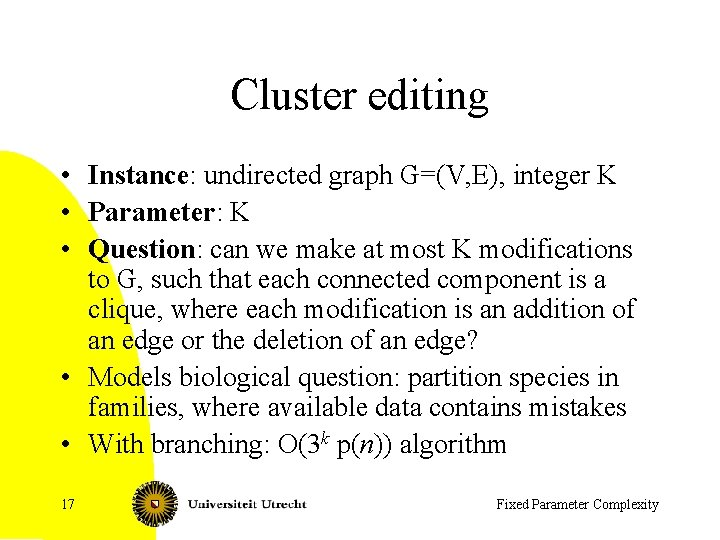Cluster editing • Instance: undirected graph G=(V, E), integer K • Parameter: K • Question: can we make at most K modifications to G, such that each connected component is a clique, where each modification is an addition of an edge or the deletion of an edge? • Models biological question: partition species in families, where available data contains mistakes • With branching: O(3 k p(n)) algorithm 17 Fixed Parameter Complexity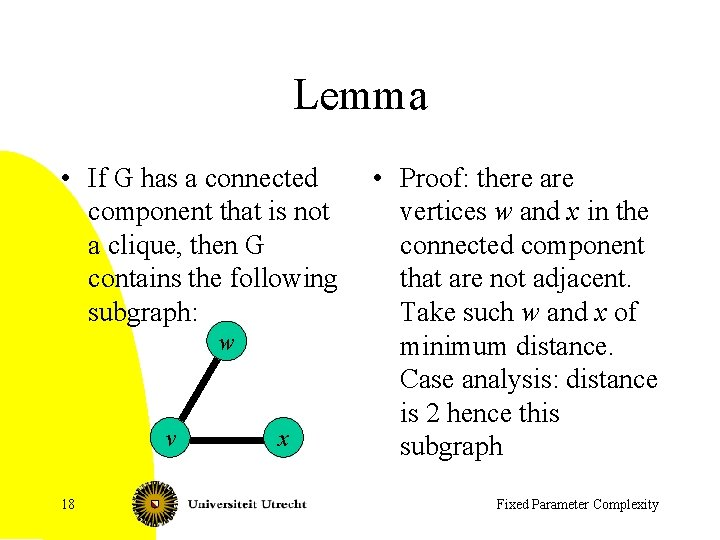Lemma • If G has a connected component that is not a clique, then G contains the following subgraph: w v 18 x • Proof: there are vertices w and x in the connected component that are not adjacent. Take such w and x of minimum distance. Case analysis: distance is 2 hence this subgraph Fixed Parameter Complexity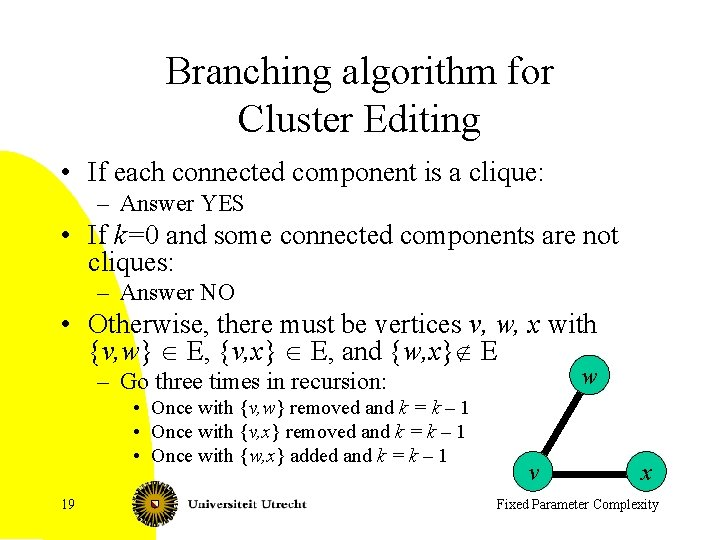Branching algorithm for Cluster Editing • If each connected component is a clique: – Answer YES • If k=0 and some connected components are not cliques: – Answer NO • Otherwise, there must be vertices v, w, x with {v, w} Î E, {v, x} Î E, and {w, x}Ï E w – Go three times in recursion: • Once with {v, w} removed and k = k – 1 • Once with {v, x} removed and k = k – 1 • Once with {w, x} added and k = k – 1 19 v x Fixed Parameter Complexity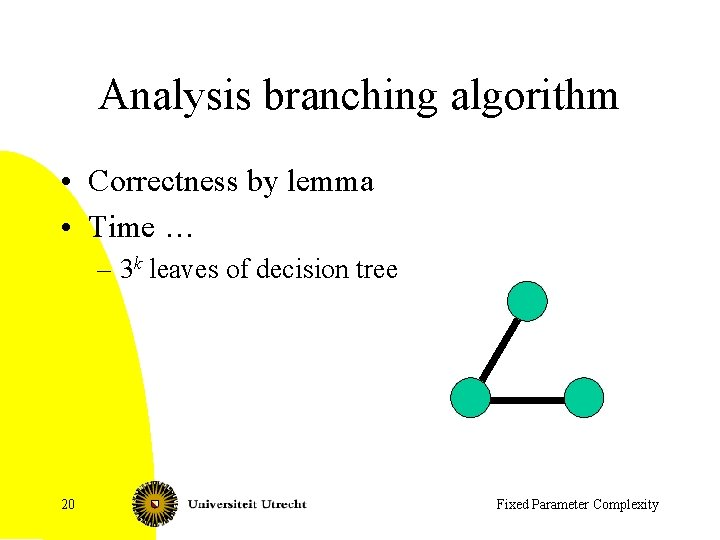Analysis branching algorithm • Correctness by lemma • Time … – 3 k leaves of decision tree 20 Fixed Parameter Complexity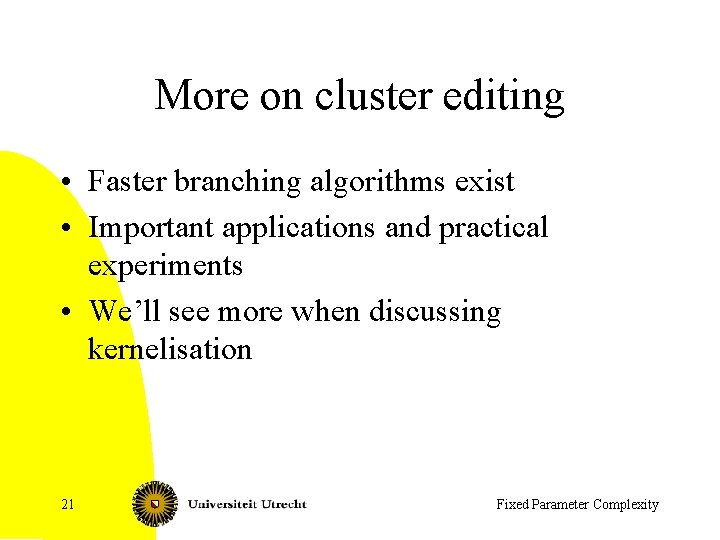More on cluster editing • Faster branching algorithms exist • Important applications and practical experiments • We’ll see more when discussing kernelisation 21 Fixed Parameter Complexity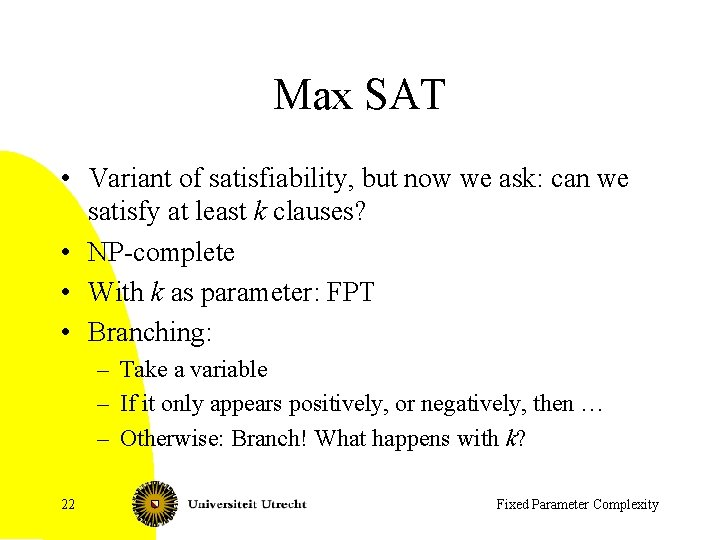Max SAT • Variant of satisfiability, but now we ask: can we satisfy at least k clauses? • NP-complete • With k as parameter: FPT • Branching: – Take a variable – If it only appears positively, or negatively, then … – Otherwise: Branch! What happens with k? 22 Fixed Parameter Complexity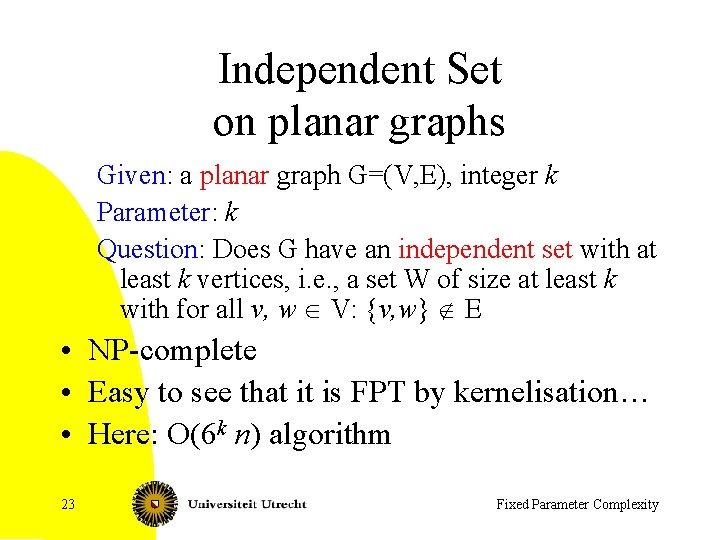Independent Set on planar graphs Given: a planar graph G=(V, E), integer k Parameter: k Question: Does G have an independent set with at least k vertices, i. e. , a set W of size at least k with for all v, w Î V: {v, w} Ï E • NP-complete • Easy to see that it is FPT by kernelisation… • Here: O(6 k n) algorithm 23 Fixed Parameter ComplexityThe red vertices form an independent set 24 Fixed Parameter ComplexityBranching • Each planar graph has a vertex of degree at most 5 • Take vertex v of minimum degree, say with neighbors w 1, …, wr, r at most 5 • A maximum size independent set contains v or one of its neighbors – Selecting a vertex is equivalent to removing it and its neighbors and decreasing k by one • Create at most 6 subproblems, one for each x Î {v, w 1, …, wr}. In each, we set k = k – 1, and remove x and its neighbors 25 Fixed Parameter Complexityv w 1 w 2 26 Fixed Parameter Complexity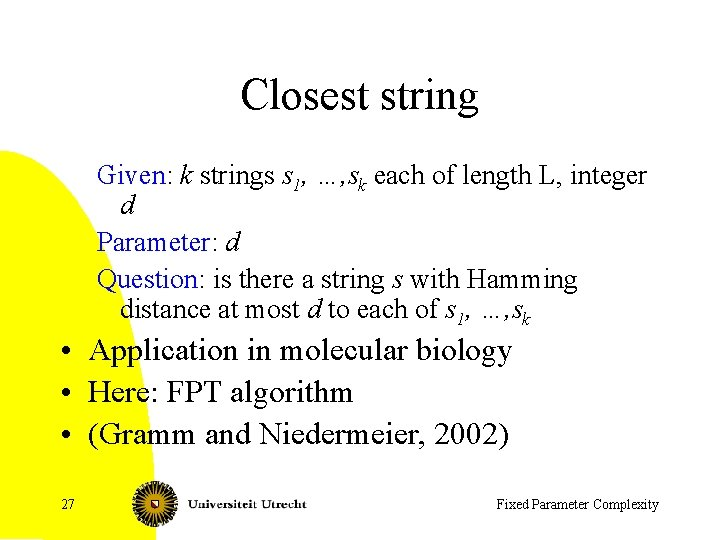Closest string Given: k strings s 1, …, sk each of length L, integer d Parameter: d Question: is there a string s with Hamming distance at most d to each of s 1, …, sk • Application in molecular biology • Here: FPT algorithm • (Gramm and Niedermeier, 2002) 27 Fixed Parameter Complexity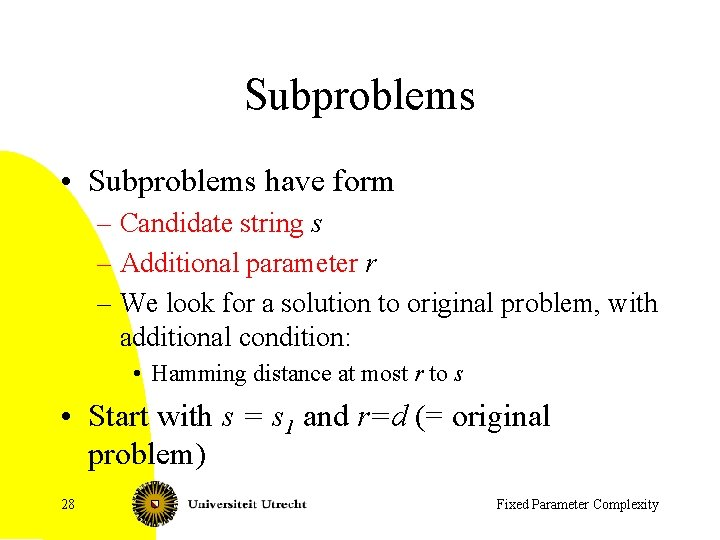Subproblems • Subproblems have form – Candidate string s – Additional parameter r – We look for a solution to original problem, with additional condition: • Hamming distance at most r to s • Start with s = s 1 and r=d (= original problem) 28 Fixed Parameter ComplexityBranching step • Choose an sj with Hamming distance > d to s • If Hamming distance of sj to s is larger than d+r: NO • For all positions i where sj differs from s – Solve subproblem with • s changed at position i to value sj (i) • r=r– 1 • Note: we find a solution, if and only one of these subproblems has a solution 29 Fixed Parameter Complexity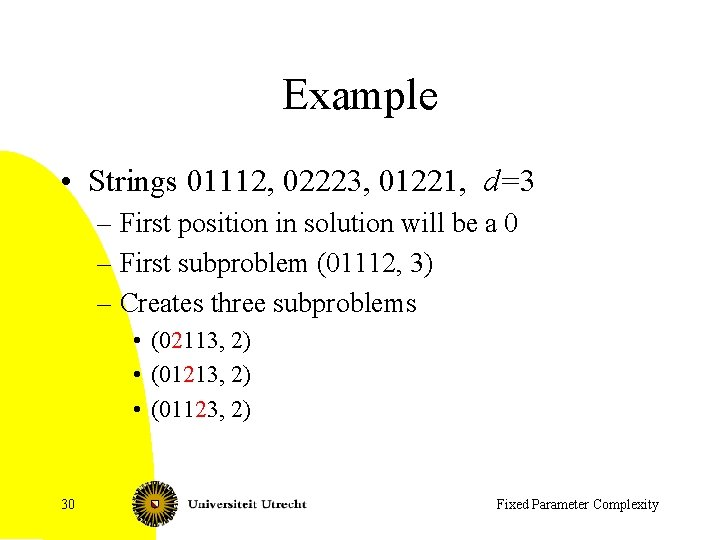Example • Strings 01112, 02223, 01221, d=3 – First position in solution will be a 0 – First subproblem (01112, 3) – Creates three subproblems • (02113, 2) • (01213, 2) • (01123, 2) 30 Fixed Parameter Complexity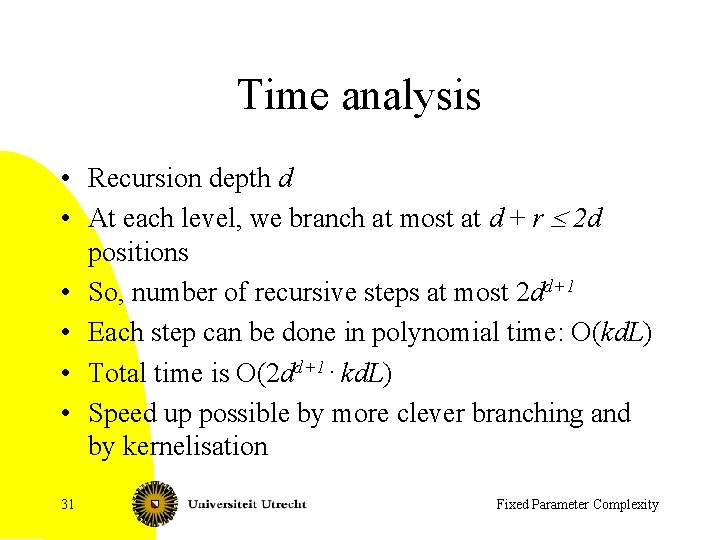Time analysis • Recursion depth d • At each level, we branch at most at d + r £ 2 d positions • So, number of recursive steps at most 2 dd+1 • Each step can be done in polynomial time: O(kd. L) • Total time is O(2 dd+1. kd. L) • Speed up possible by more clever branching and by kernelisation 31 Fixed Parameter Complexity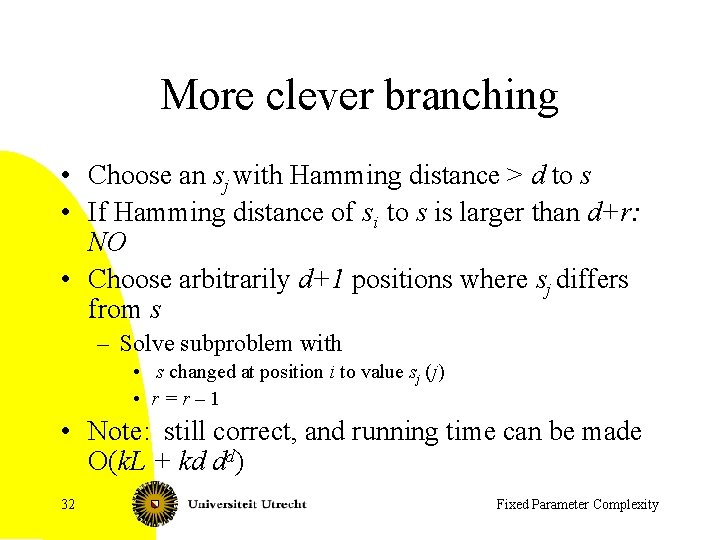More clever branching • Choose an sj with Hamming distance > d to s • If Hamming distance of si to s is larger than d+r: NO • Choose arbitrarily d+1 positions where sj differs from s – Solve subproblem with • s changed at position i to value sj (j) • r=r– 1 • Note: still correct, and running time can be made O(k. L + kd dd) 32 Fixed Parameter Complexity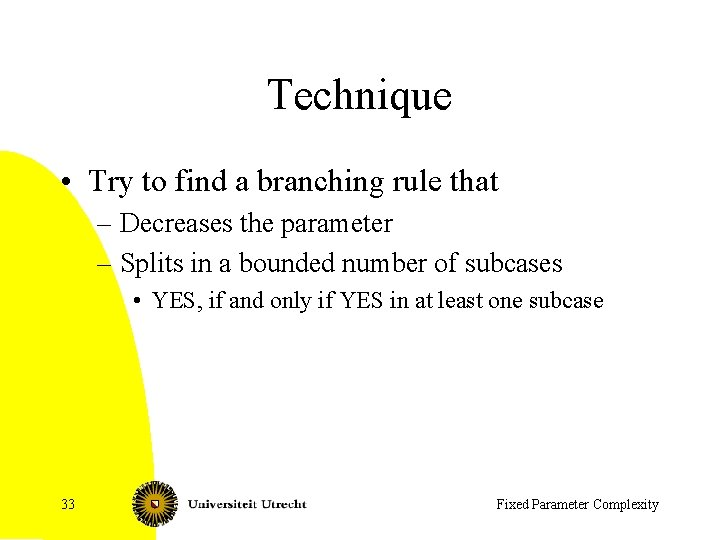Technique • Try to find a branching rule that – Decreases the parameter – Splits in a bounded number of subcases • YES, if and only if YES in at least one subcase 33 Fixed Parameter ComplexityKernelisation • Preprocessing rules reduce starting instance to one of size f(k) – Should work in polynomial time • Then use any algorithm to solve problem on kernel • Time will be p(n) + g(f(k)) 34 Fixed Parameter Complexity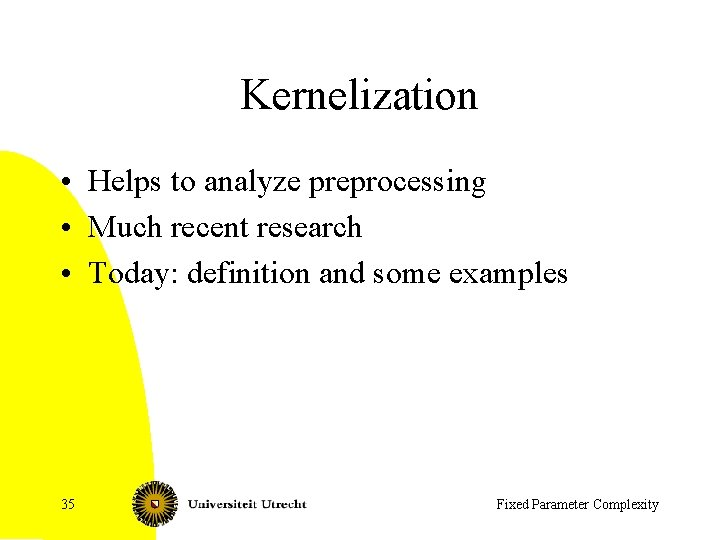Kernelization • Helps to analyze preprocessing • Much recent research • Today: definition and some examples 35 Fixed Parameter Complexity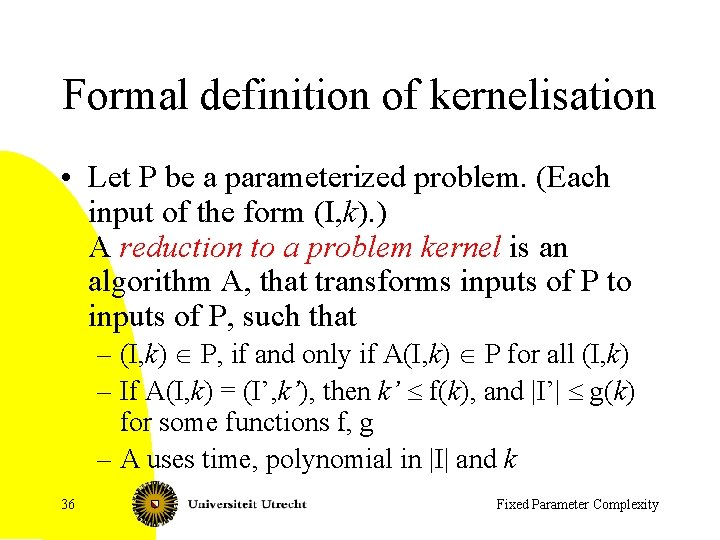Formal definition of kernelisation • Let P be a parameterized problem. (Each input of the form (I, k). ) A reduction to a problem kernel is an algorithm A, that transforms inputs of P to inputs of P, such that – (I, k) Î P, if and only if A(I, k) Î P for all (I, k) – If A(I, k) = (I’, k’), then k’ £ f(k), and |I’| £ g(k) for some functions f, g – A uses time, polynomial in |I| and k 36 Fixed Parameter ComplexityKernels and FPT • Theorem. Consider a decidable parameterized problem. Then the problem belongs to FPT, if and only if it has a kernel • <= Build the kernel and then solve the problem on the kernel • => Suppose we have an f(k)nc algorithm. Run the algorithm for nc+1 steps. If it did not yet solve the problem, return the input as kernel: it has size at most f(k). If it solved the problem, then … 37 Fixed Parameter Complexity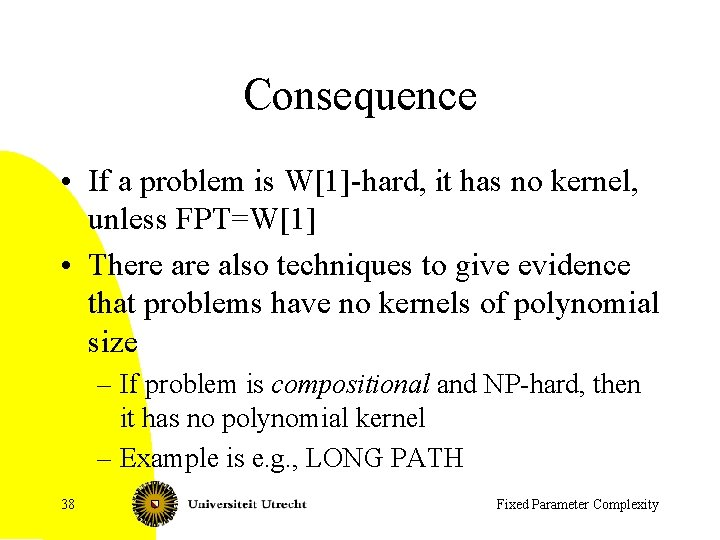Consequence • If a problem is W-hard, it has no kernel, unless FPT=W • There also techniques to give evidence that problems have no kernels of polynomial size – If problem is compositional and NP-hard, then it has no polynomial kernel – Example is e. g. , LONG PATH 38 Fixed Parameter Complexity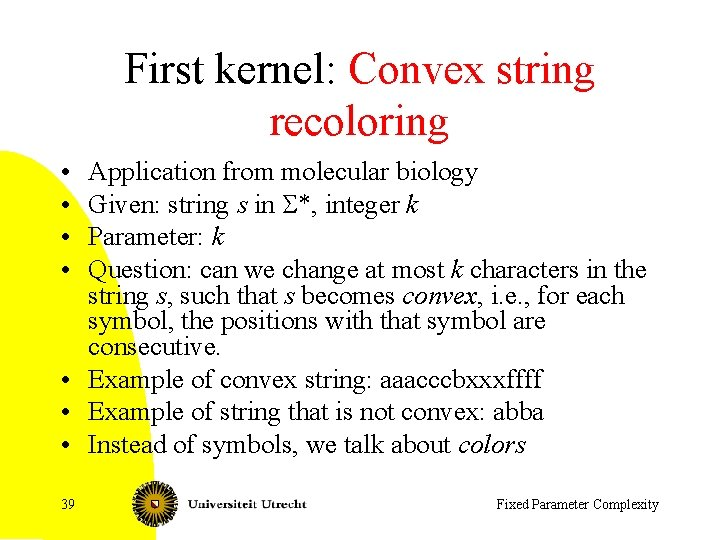First kernel: Convex string recoloring • • Application from molecular biology Given: string s in S*, integer k Parameter: k Question: can we change at most k characters in the string s, such that s becomes convex, i. e. , for each symbol, the positions with that symbol are consecutive. • Example of convex string: aaacccbxxxffff • Example of string that is not convex: abba • Instead of symbols, we talk about colors 39 Fixed Parameter Complexity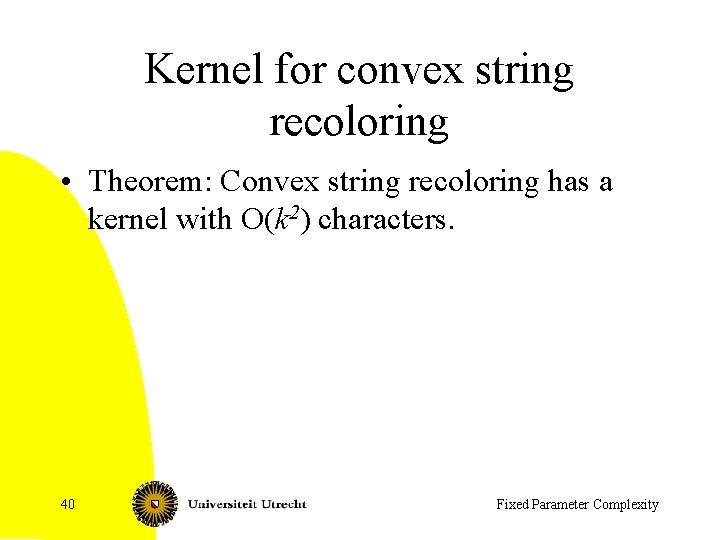Kernel for convex string recoloring • Theorem: Convex string recoloring has a kernel with O(k 2) characters. 40 Fixed Parameter ComplexityNotions • Notion: good and bad colors • A color is good, if it is consecutive in s, otherwise it is bad • abba: a is bad and b is good • Notion: block: consecutive occurrences of the same color: aaabbbaccc has four blocks • Convex: each color has one block 41 Fixed Parameter Complexity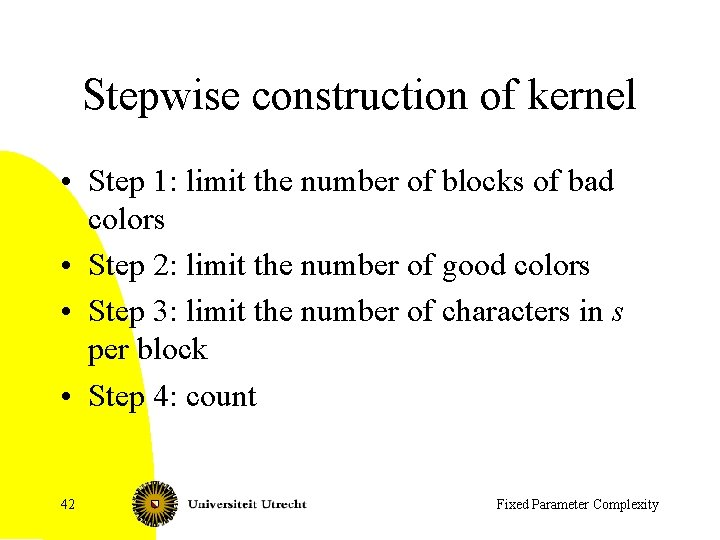Stepwise construction of kernel • Step 1: limit the number of blocks of bad colors • Step 2: limit the number of good colors • Step 3: limit the number of characters in s per block • Step 4: count 42 Fixed Parameter Complexity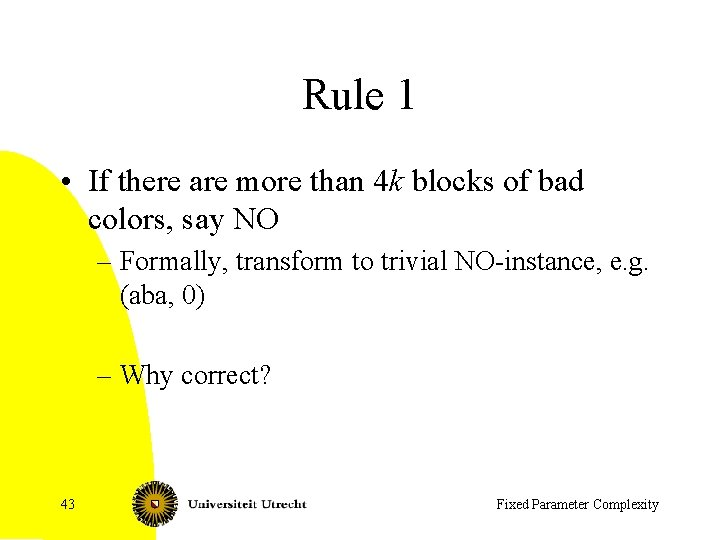Rule 1 • If there are more than 4 k blocks of bad colors, say NO – Formally, transform to trivial NO-instance, e. g. (aba, 0) – Why correct? 43 Fixed Parameter Complexity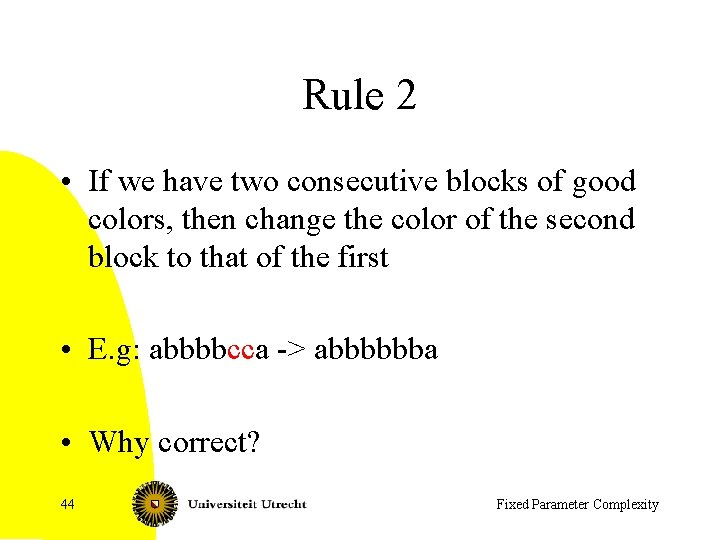Rule 2 • If we have two consecutive blocks of good colors, then change the color of the second block to that of the first • E. g: abbbbcca -> abbbbbba • Why correct? 44 Fixed Parameter Complexity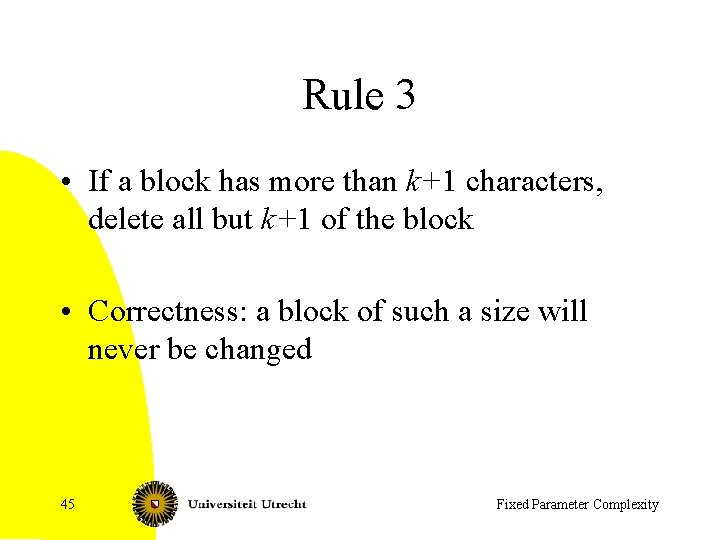Rule 3 • If a block has more than k+1 characters, delete all but k+1 of the block • Correctness: a block of such a size will never be changed 45 Fixed Parameter ComplexityCounting • After the rules have been applied, we have at most: – 4 k blocks of bad colors – 4 k+1 blocks of good colors: at most one between each pair of bad colors, one in front and one in the end – Each block has size at most k+1 • String has size at most (8 k+1)(k+1) • This can be improved by better analysis, more rules, . . . 46 Fixed Parameter ComplexityVertex cover: observations that helps for kernelisation • If v has degree at least k+1, then v belongs to each vertex cover in G of size at most k. – If v is not in the vertex cover, then all its neighbors are in the vertex cover. • If all vertices have degree at most k, then a vertex cover has at least m/k vertices. – (m=|E|). Any vertex covers at most k edges. 47 Fixed Parameter ComplexityKernelisation for Vertex Cover H = G; ( S = Æ; ) While there is a vertex v in H of degree at least k+1 do Remove v and its incident edges from H k = k – 1; ( S = S + v ; ) If k < 0 then return false If H has at least k 2+1 edges, then return false Remove vertices of degree 0 Solve vertex cover on (H, k) with some algorithm 48 Fixed Parameter Complexity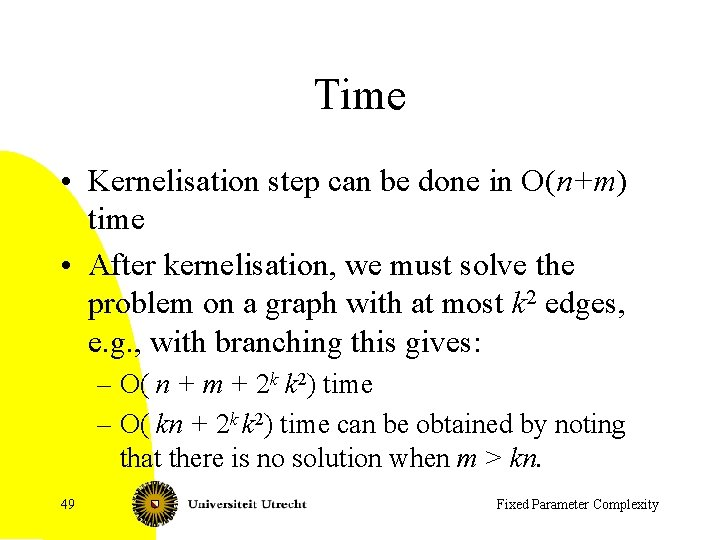Time • Kernelisation step can be done in O(n+m) time • After kernelisation, we must solve the problem on a graph with at most k 2 edges, e. g. , with branching this gives: – O( n + m + 2 k k 2) time – O( kn + 2 k k 2) time can be obtained by noting that there is no solution when m > kn. 49 Fixed Parameter ComplexityBetter kernel for vertex cover • • • Nemhauser-Trotter: kernel of at most 2 k vertices Make ILP formulation of Vertex Cover Solve relaxation All vertices v with xv > ½ : put v in set All vertices v with xv < ½ : v is not in the set Remove all vertices except those with value ½, and decrease k accordingly • Gives kernel with at most 2 k vertices, but why is it correct? 50 Fixed Parameter Complexity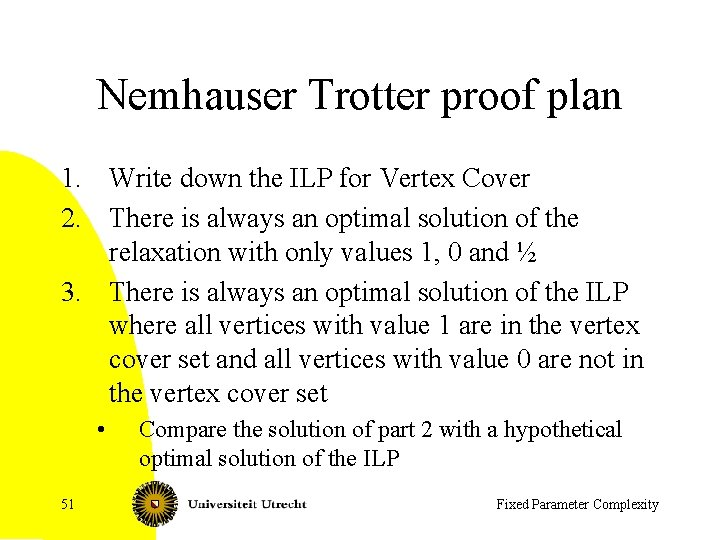Nemhauser Trotter proof plan 1. Write down the ILP for Vertex Cover 2. There is always an optimal solution of the relaxation with only values 1, 0 and ½ 3. There is always an optimal solution of the ILP where all vertices with value 1 are in the vertex cover set and all vertices with value 0 are not in the vertex cover set • 51 Compare the solution of part 2 with a hypothetical optimal solution of the ILP Fixed Parameter Complexity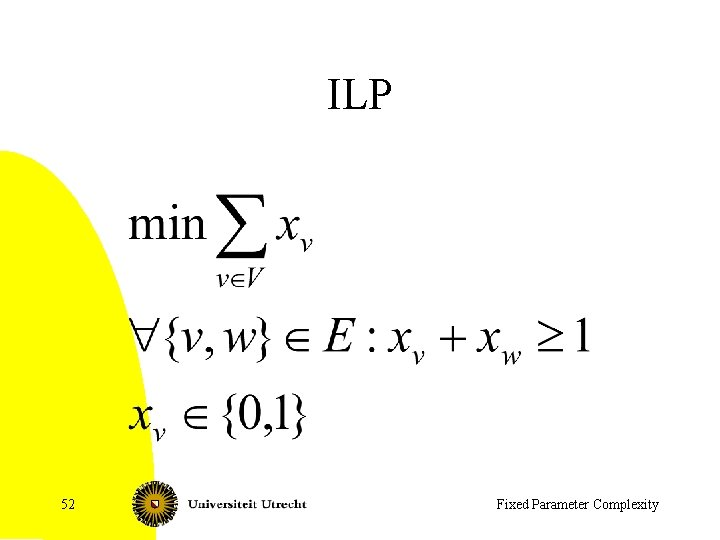ILP 52 Fixed Parameter Complexity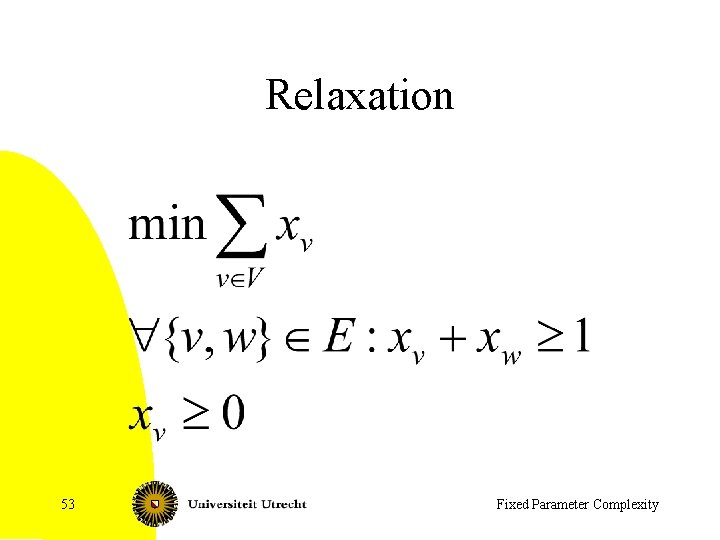Relaxation 53 Fixed Parameter Complexity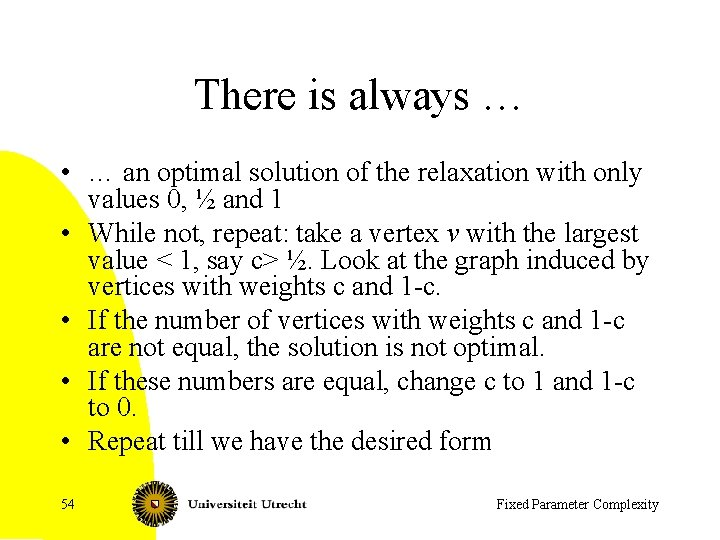There is always … • … an optimal solution of the relaxation with only values 0, ½ and 1 • While not, repeat: take a vertex v with the largest value < 1, say c> ½. Look at the graph induced by vertices with weights c and 1 -c. • If the number of vertices with weights c and 1 -c are not equal, the solution is not optimal. • If these numbers are equal, change c to 1 and 1 -c to 0. • Repeat till we have the desired form 54 Fixed Parameter ComplexityVertices with weight 0 and 1 • There is an optimal solution of the ILP with vertices with weight 0 and weight 1 in the relaxation not changed • A = weight in relaxation 1 • B = weight in relaxation 0 • C = weight in relaxation ½ • Note: no edges from B to C • Take ILP solution x and relation y – Follow x on C and y on A and B: this is a solution – It is optimal, otherwise, taking y on C and x on A and B was not an optimal solution for the relaxation 55 Fixed Parameter Complexity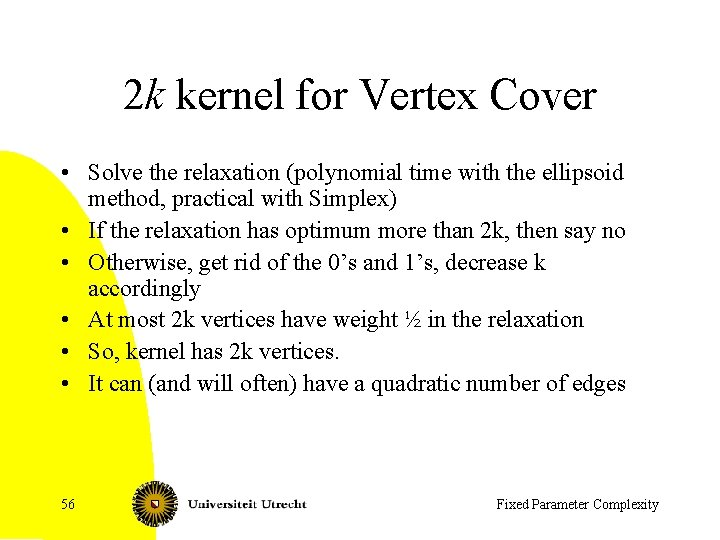2 k kernel for Vertex Cover • Solve the relaxation (polynomial time with the ellipsoid method, practical with Simplex) • If the relaxation has optimum more than 2 k, then say no • Otherwise, get rid of the 0’s and 1’s, decrease k accordingly • At most 2 k vertices have weight ½ in the relaxation • So, kernel has 2 k vertices. • It can (and will often) have a quadratic number of edges 56 Fixed Parameter Complexity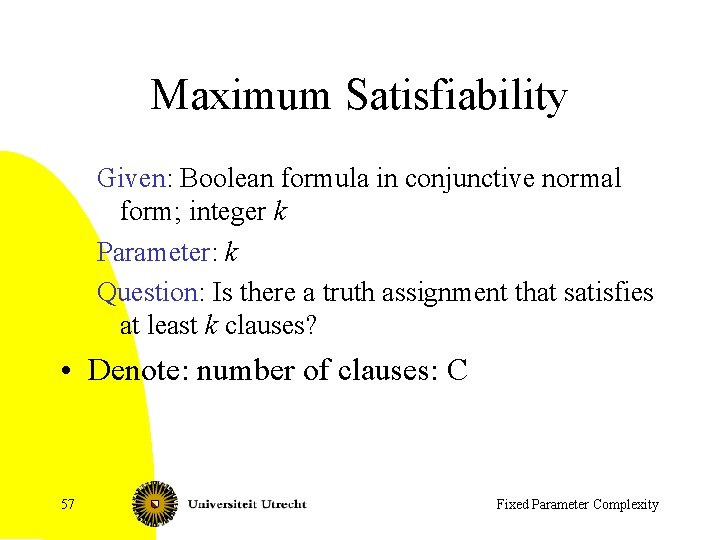Maximum Satisfiability Given: Boolean formula in conjunctive normal form; integer k Parameter: k Question: Is there a truth assignment that satisfies at least k clauses? • Denote: number of clauses: C 57 Fixed Parameter Complexity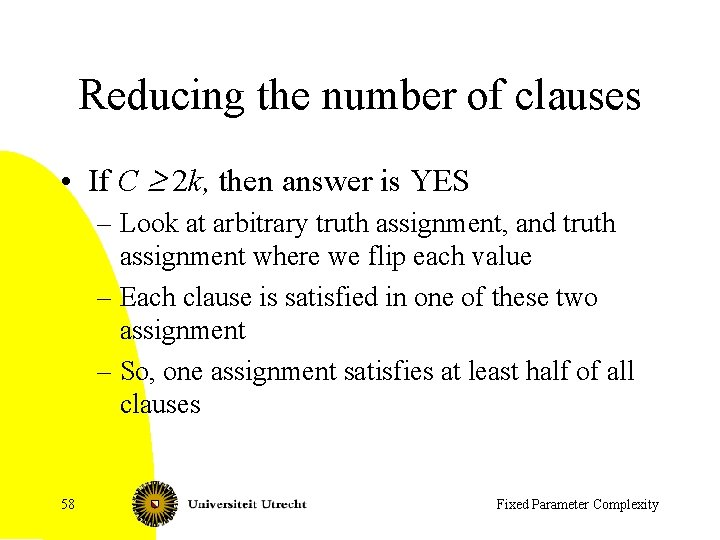Reducing the number of clauses • If C ³ 2 k, then answer is YES – Look at arbitrary truth assignment, and truth assignment where we flip each value – Each clause is satisfied in one of these two assignment – So, one assignment satisfies at least half of all clauses 58 Fixed Parameter ComplexityBounding number of long clauses • • Long clause: has at least k literals Short clause: has at most k-1 literals Let L be number of long clauses If L ³ k: answer is YES – Select in each long clause a literal, whose complement is not yet selected – Set these all to true – All long clauses are satisfied 59 Fixed Parameter Complexity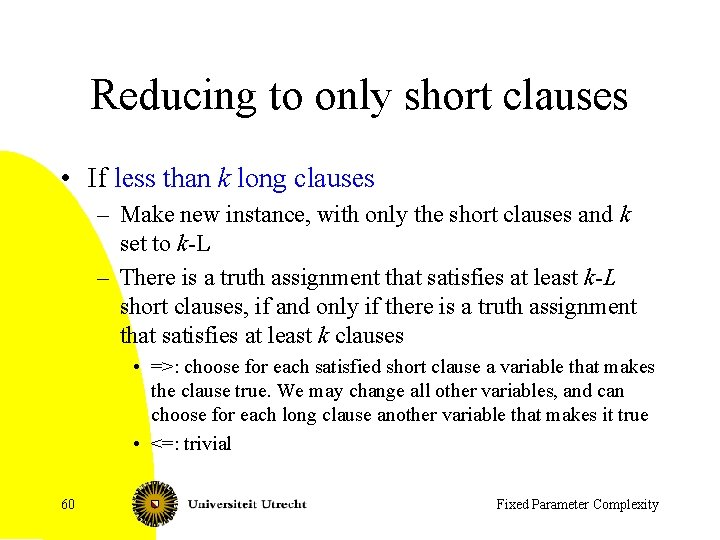Reducing to only short clauses • If less than k long clauses – Make new instance, with only the short clauses and k set to k-L – There is a truth assignment that satisfies at least k-L short clauses, if and only if there is a truth assignment that satisfies at least k clauses • =>: choose for each satisfied short clause a variable that makes the clause true. We may change all other variables, and can choose for each long clause another variable that makes it true • <=: trivial 60 Fixed Parameter Complexity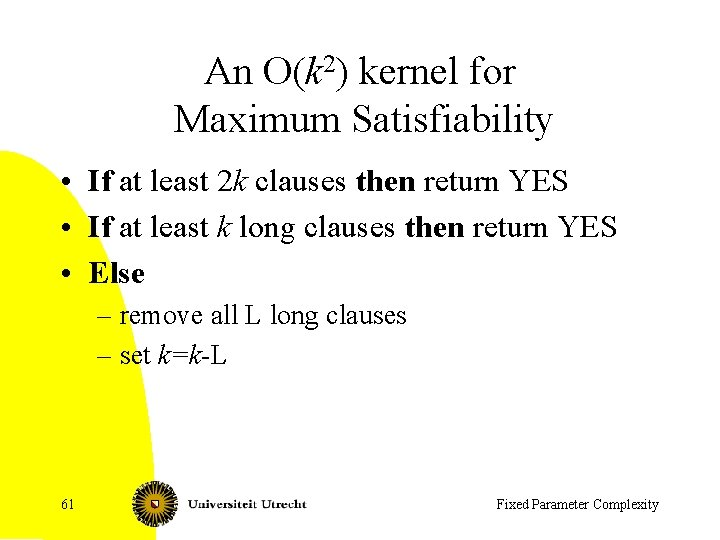An O(k 2) kernel for Maximum Satisfiability • If at least 2 k clauses then return YES • If at least k long clauses then return YES • Else – remove all L long clauses – set k=k-L 61 Fixed Parameter Complexity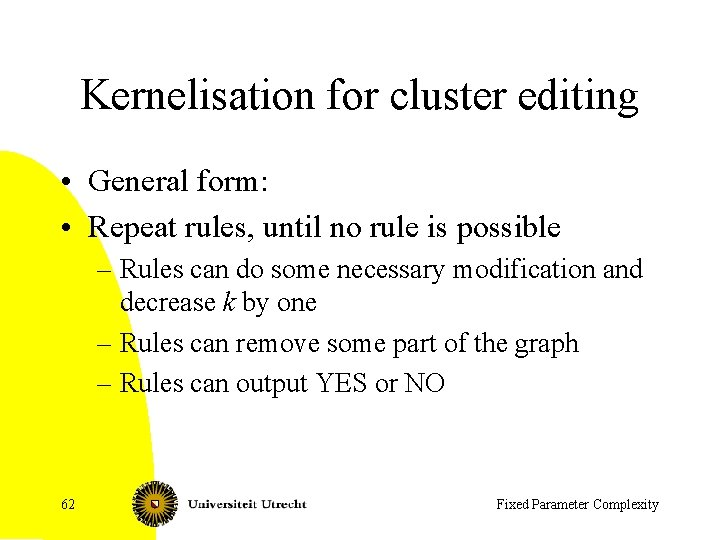Kernelisation for cluster editing • General form: • Repeat rules, until no rule is possible – Rules can do some necessary modification and decrease k by one – Rules can remove some part of the graph – Rules can output YES or NO 62 Fixed Parameter ComplexityTrivial rules and plan • Rule 1: If a connected component of G is a clique, remove this connected component • Rule 2: If we have more than k connected components and Rule 1 does not apply: Answer NO • Consequence: after Rule 1 and Rule 2, there at most k connected component • Plan: find rules that make connected component small • We change the input: some pairs are permanent and others are forbidden. 63 Fixed Parameter ComplexityObservation and rule 3 • If two vertices v, w have k+1 neighbors in common, they must belong to the same clique in a solution – If the edge did not exist, add it and decrease k by 1 – Set the edge {v, w} to be permanent 64 Fixed Parameter Complexity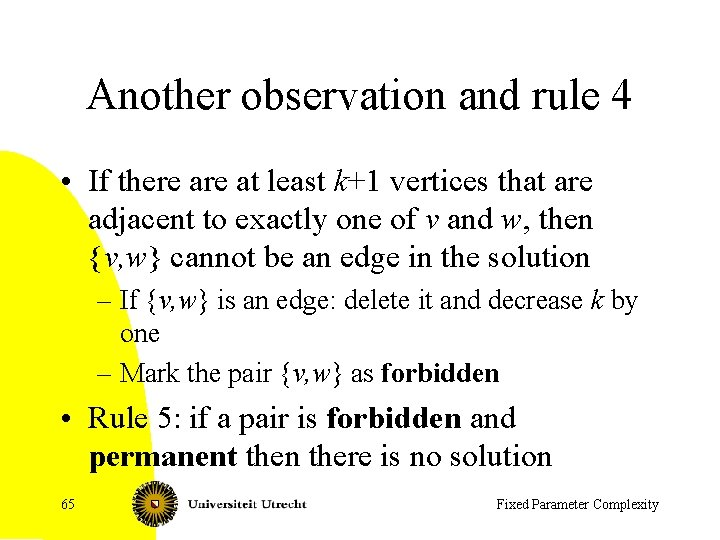Another observation and rule 4 • If there at least k+1 vertices that are adjacent to exactly one of v and w, then {v, w} cannot be an edge in the solution – If {v, w} is an edge: delete it and decrease k by one – Mark the pair {v, w} as forbidden • Rule 5: if a pair is forbidden and permanent then there is no solution 65 Fixed Parameter Complexity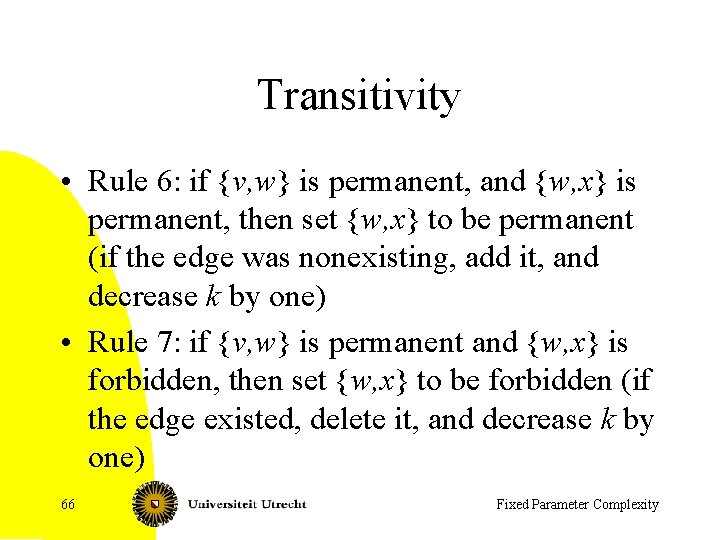Transitivity • Rule 6: if {v, w} is permanent, and {w, x} is permanent, then set {w, x} to be permanent (if the edge was nonexisting, add it, and decrease k by one) • Rule 7: if {v, w} is permanent and {w, x} is forbidden, then set {w, x} to be forbidden (if the edge existed, delete it, and decrease k by one) 66 Fixed Parameter Complexity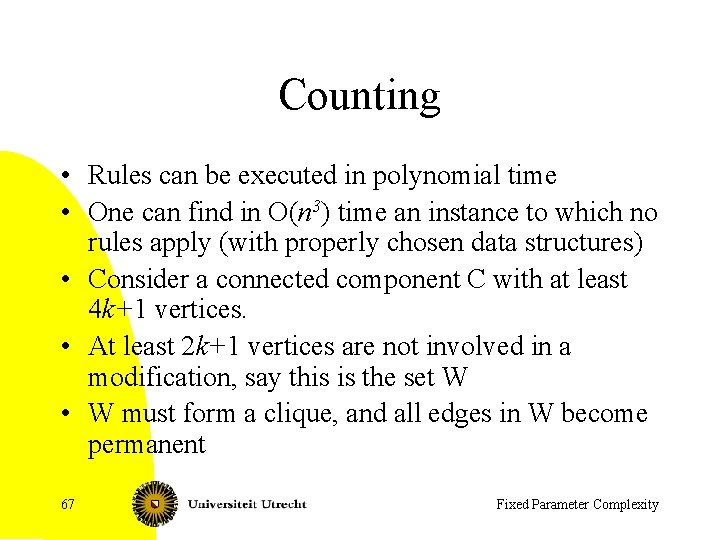Counting • Rules can be executed in polynomial time • One can find in O(n 3) time an instance to which no rules apply (with properly chosen data structures) • Consider a connected component C with at least 4 k+1 vertices. • At least 2 k+1 vertices are not involved in a modification, say this is the set W • W must form a clique, and all edges in W become permanent 67 Fixed Parameter ComplexityCounting continued • Each vertex in C-W that is incident to k+1 or more vertices in W has a permanent edge to a vertex in W, and then gets permanent edges to all vertices in W, and then becomes member of W • Each vertex in C-W for which at least k+1 vertices in W are not adjacent: it gets a forbidden edge to each vertex in W • Each vertex in C-W is handled as |W|>2 k. • So, each connected component has size at most 4 k • In total at most 4 k 2 vertices 68 Fixed Parameter ComplexityComments • This argument is due to Gramm et al. • Better and more recent algorithms exist: faster branching (2. 7 k) and linear kernels 69 Fixed Parameter ComplexityNon-blocker • Given: graph G=(V, E), integer k • Parameter k • Question: Does G have a dominating set of size at most |V|-k ? 70 Fixed Parameter Complexity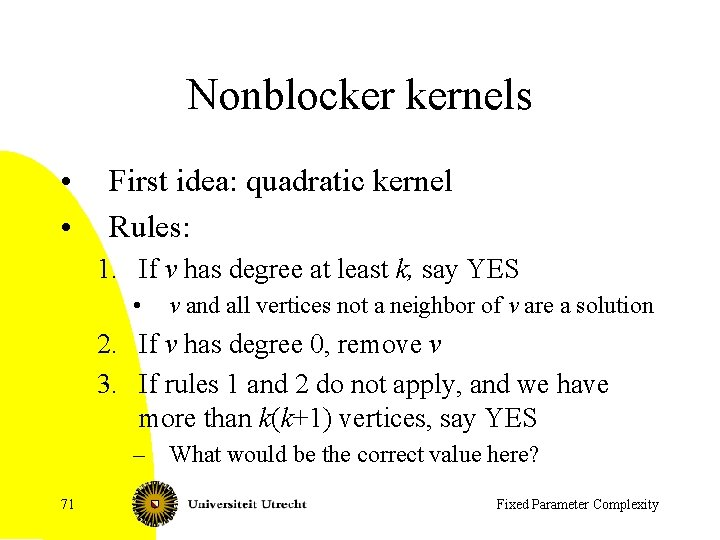Nonblocker kernels • • First idea: quadratic kernel Rules: 1. If v has degree at least k, say YES • v and all vertices not a neighbor of v are a solution 2. If v has degree 0, remove v 3. If rules 1 and 2 do not apply, and we have more than k(k+1) vertices, say YES – What would be the correct value here? 71 Fixed Parameter Complexity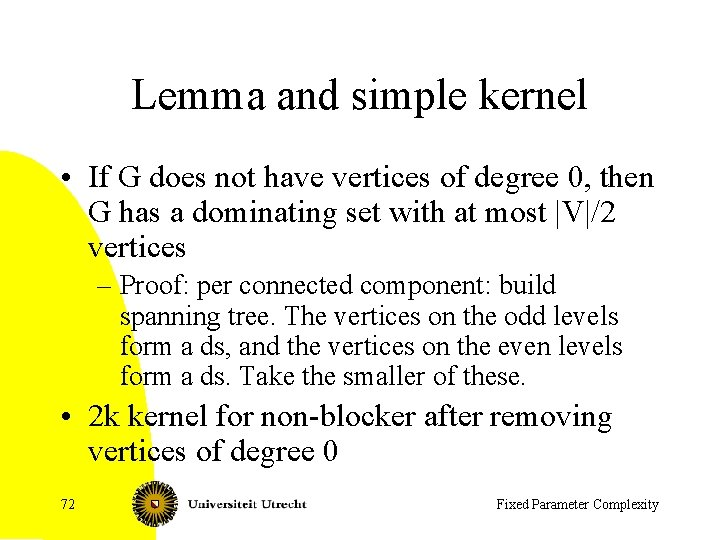Lemma and simple kernel • If G does not have vertices of degree 0, then G has a dominating set with at most |V|/2 vertices – Proof: per connected component: build spanning tree. The vertices on the odd levels form a ds, and the vertices on the even levels form a ds. Take the smaller of these. • 2 k kernel for non-blocker after removing vertices of degree 0 72 Fixed Parameter Complexity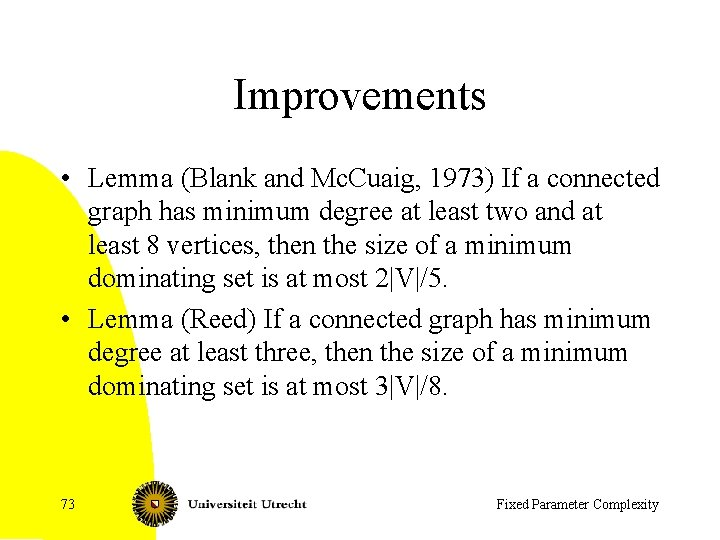Improvements • Lemma (Blank and Mc. Cuaig, 1973) If a connected graph has minimum degree at least two and at least 8 vertices, then the size of a minimum dominating set is at most 2|V|/5. • Lemma (Reed) If a connected graph has minimum degree at least three, then the size of a minimum dominating set is at most 3|V|/8. 73 Fixed Parameter ComplexityGetting rid of vertices of degree 1 • Idea: we get rid of all but one vertices of degree 1 • Rule: if v and w have degree 1, then identify the neighbor of v and w and remove w • Intuition: we can assume that a neighbor of a vertex of degree 1 is in the dominating set • Possibly, replace in the end vertex of degree 1 by triangle 74 Fixed Parameter ComplexityDegree two • If we have an induced path like this: x y • Then remove the two middle vertices and identify the endpoints 75 Fixed Parameter Complexity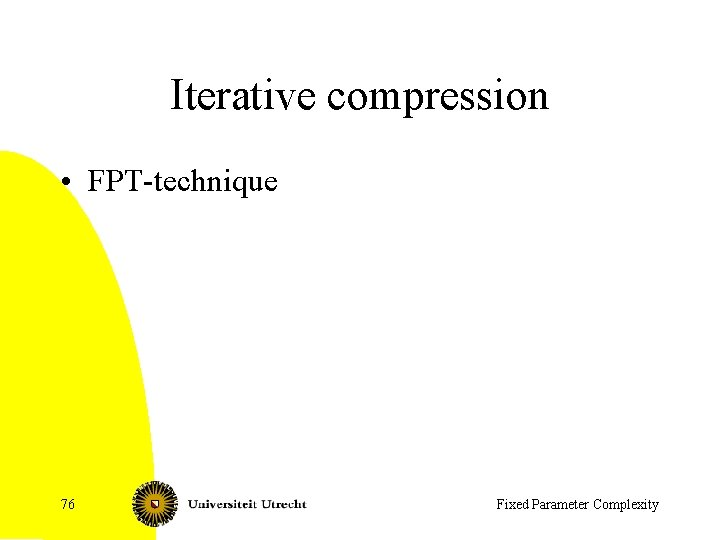Iterative compression • FPT-technique 76 Fixed Parameter ComplexityFeedback Vertex Set • Instance: graph G=(V, E) • Parameter: integer k • Question: Is there a set of at most k vertices W, such that G-W is a forest? – Known in FPT – Here: recent algorithm O(5 k p(n)) time algorithm – Can be done in O(5 k kn) or less with kernelisation 77 Fixed Parameter Complexity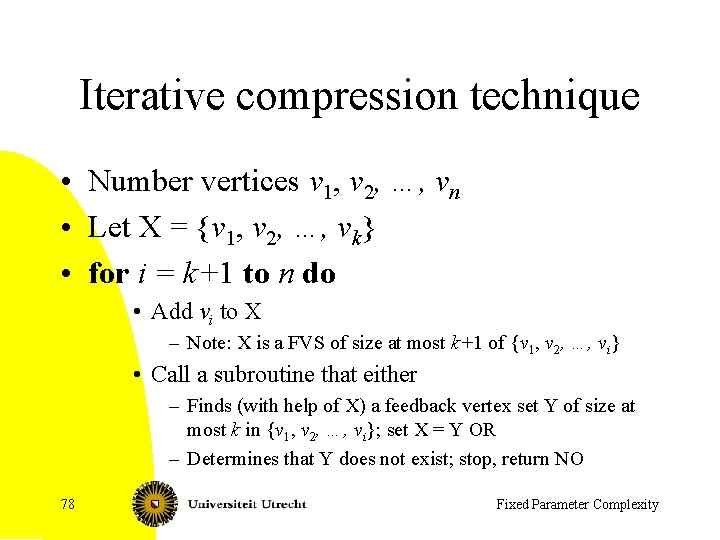Iterative compression technique • Number vertices v 1, v 2, …, vn • Let X = {v 1, v 2, …, vk} • for i = k+1 to n do • Add vi to X – Note: X is a FVS of size at most k+1 of {v 1, v 2, …, vi} • Call a subroutine that either – Finds (with help of X) a feedback vertex set Y of size at most k in {v 1, v 2, …, vi}; set X = Y OR – Determines that Y does not exist; stop, return NO 78 Fixed Parameter Complexity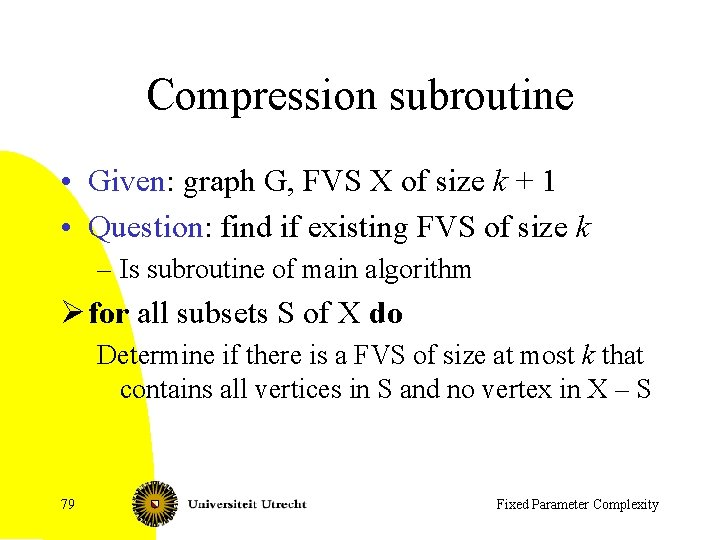Compression subroutine • Given: graph G, FVS X of size k + 1 • Question: find if existing FVS of size k – Is subroutine of main algorithm Ø for all subsets S of X do Determine if there is a FVS of size at most k that contains all vertices in S and no vertex in X – S 79 Fixed Parameter Complexity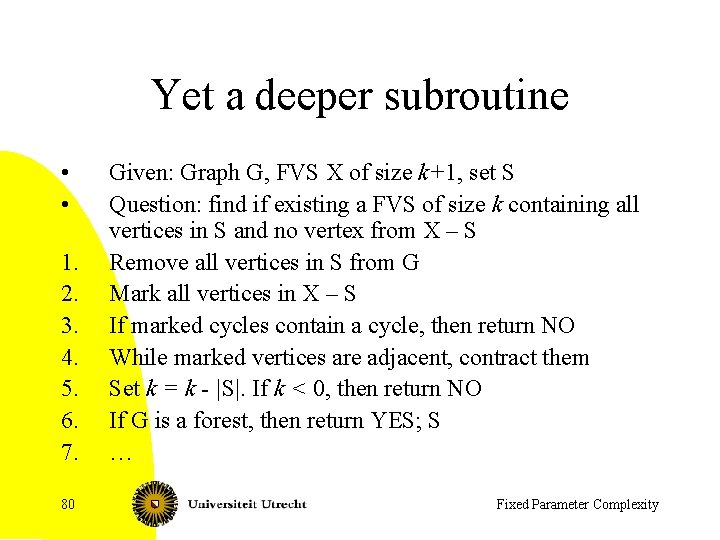Yet a deeper subroutine • • 1. 2. 3. 4. 5. 6. 7. 80 Given: Graph G, FVS X of size k+1, set S Question: find if existing a FVS of size k containing all vertices in S and no vertex from X – S Remove all vertices in S from G Mark all vertices in X – S If marked cycles contain a cycle, then return NO While marked vertices are adjacent, contract them Set k = k - |S|. If k < 0, then return NO If G is a forest, then return YES; S … Fixed Parameter ComplexitySubroutine continued 7. If an unmarked vertex v has at least two edges to marked vertices • If these edges are parallel, i. e. , to the same neighbor, then v must be in a FVS (we have a cycle with v the only unmarked vertex) • • Put v in S, set k = k – 1 and recurse Else recurse twice: • • Put v in S, set k = k – 1 and recurse Mark v, contract v with all marked neighbors and recurse – 81 The number of marked vertices is one smaller Fixed Parameter Complexity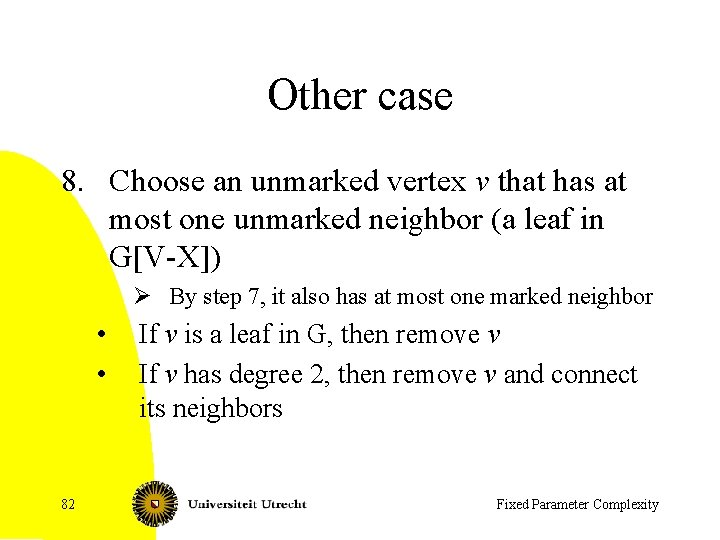Other case 8. Choose an unmarked vertex v that has at most one unmarked neighbor (a leaf in G[V-X]) Ø By step 7, it also has at most one marked neighbor • • 82 If v is a leaf in G, then remove v If v has degree 2, then remove v and connect its neighbors Fixed Parameter Complexity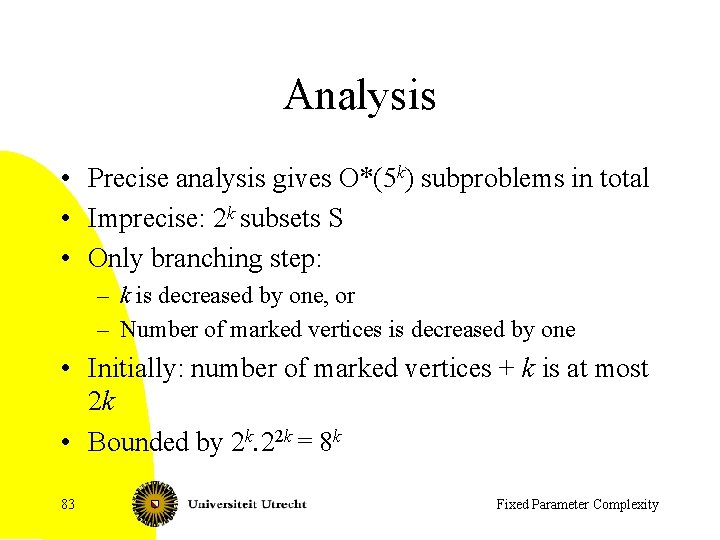Analysis • Precise analysis gives O*(5 k) subproblems in total • Imprecise: 2 k subsets S • Only branching step: – k is decreased by one, or – Number of marked vertices is decreased by one • Initially: number of marked vertices + k is at most 2 k • Bounded by 2 k. 22 k = 8 k 83 Fixed Parameter ComplexityConclusions • Similar techniques work (usually much more complicated) for many other problems • W[…]-hardness results indicate that FPTalgorithms do not exist for other problems • Note similarities and differences with exponential time algorithms 84 Fixed Parameter Complexity